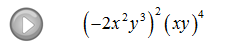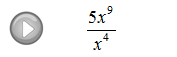## Algebra

Showing posts with label exponents. Show all posts
Showing posts with label exponents. Show all posts

### Negative Exponents

The quotient rule for exponents can be used to define negative exponents. It might seem strange to think of negative exponents, but we need to know where they come from and how to work with them.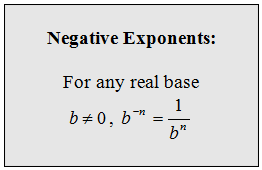Factors in the numerator with a negative exponents move to the denominator.Simplify.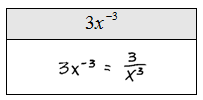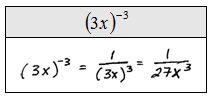If you are given a factor with a negative exponent in the denominator simply move it to the numerator. Use the following reasoning to justify this.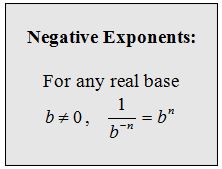Simplify.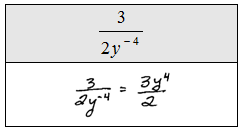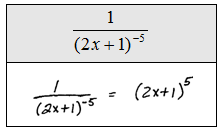A common mistake is to multiply the base with the exponent when it is negative. For example,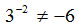Avoid this mistake . The correct solution isAnother useful property involves a rational expression raised to a negative exponent.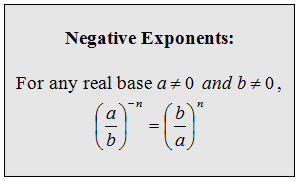Simplify.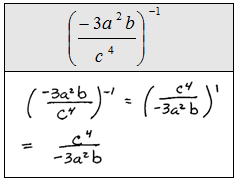When simplifying expressions, it usually is best to simplify within the parentheses first and then apply the product and/or the quotient rule.

Simplify.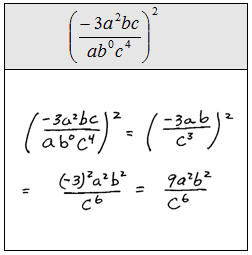Scientific notation is an application of negative exponents. It is used to express very large or very small numbers.An example of a power of ten might look like,Use this to convert number expressed in scientific notation to a decimal.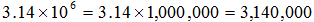Remember that we can obtain this same result by moving the decimal over six places to the right and filling in with the digit 0.

A power of ten might be negative,Negative exponents appear when working with very small numbers.Obtain the same result by moving the decimal over to the left 4 places.

Express the number in scientific notation.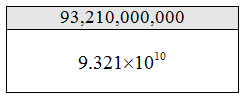Choose to place the decimal so that the first digit is between 1 and 10.

Multiplication is commutative, so when multiplying numbers in scientific notation multiply the decimal parts first. Next, multiply the powers of 10 using the product rule.

Multiply.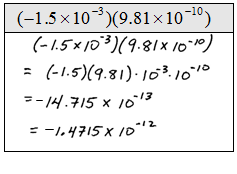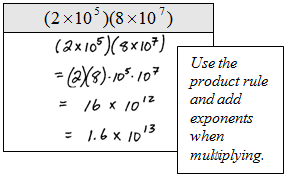Divide.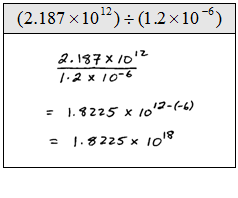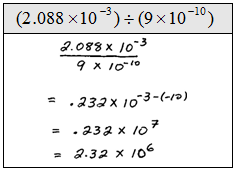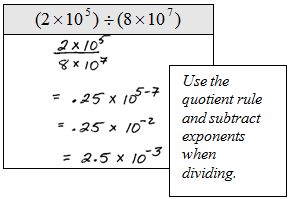### Rules of Exponents

Before learning how to add, subtract, multiply and divide polynomials we need to understand the rules of exponents.

There will be many new rules to remember and you might feel overwhelmed. However, with much practice you will quickly learn to use them effortlessly.  (In this section we will assume all variables are real and exponents are integers.)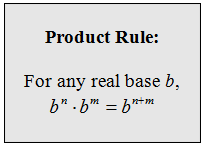When multiplying two real numbers with the same base you add exponents.Multiply.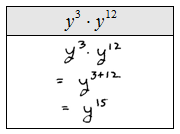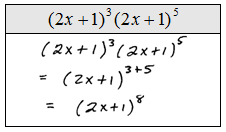Add exponents only when the bases are exactly the same.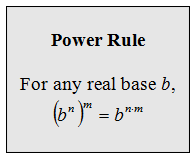When raising an exponent to a power multiply the exponents.Simplify.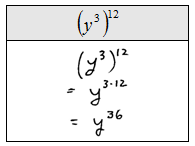Never multiply a base with an exponent.When we raise a product to a power we must raise each factor to that power.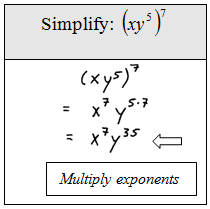Simplify.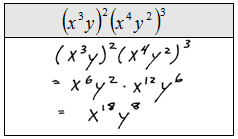Next we consider the power of a quotient.When raising a quotient to a power, raise numerator and denominator to that power. Assume the variables in the denominator are nonzero. If we have zero in the denominator then the expression is undefined.Simplify.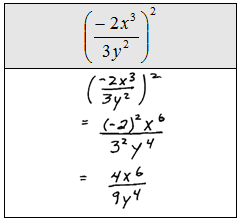If you raise expressions to an even power then the quantity will be positive. If you raise negative expressions to an odd power then the quantity will be negative.When dividing two expressions with the same base we subtract exponents. This rule is basically cancellation in disguise.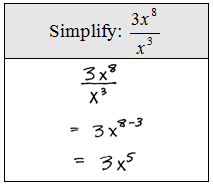Simplify (Assume all variables are nonzero.)Next we cover the zero exponent rule.When an expression is raised to the zero power it will be equal to 1, unless the base is zero. Zero to the zero power is undefined.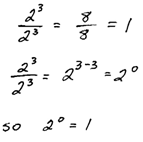Simplify (Assume all variables are nonzero.)